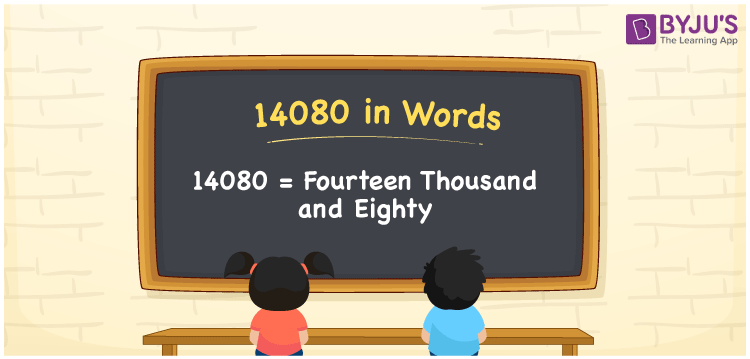# 14080 in Words

We can write 14080 in words as Fourteen thousand and eighty. When you receive an invoice of Rs. 14080 for online shopping, you can communicate the same as, “I got an invoice of Fourteen thousand and eighty rupees for online shopping”. Similarly, we can use the word form of the number 14080 in many general conversations. Let’s learn how to write 14080 using a place value here in this article.

 14080 in words Fourteen thousand and eighty Fourteen thousand and eighty in Numbers 14080

## 14080 in English Words

In general, we use the English alphabet to express numbers in words and so, we can spell 14080 in English as “Fourteen thousand and eighty”.## How to Write 14080 in Words?

In this section, you will understand how to derive the number 14080 to word form using a place value chart. The below table shows the place values for the number 14080.

 Ten thousand Thousands Hundreds Tens Ones 1 4 0 8 0

Here, ones = 0, tens = 8, hundreds = 0, thousands = 4, ten thousand = 1

So, 1 × Ten thousand + 4 × Thousand + 0 × Hundred + 8 × Ten + 0 × One

= 1 × 10000 + 4 × 1000 + 0 × 100 + 8 × 10 + 0 × 1

= 10000 + 4000 + 80

= Ten Thousand + Four thousand + Eighty

= Fourteen thousand + Eighty

= Fourteen thousand and eighty

14080 is a natural number that is the successor of 14079 and predecessor of 14081.

14080 in words – Fourteen thousand and eighty

Is 14080 an odd number? – No

Is 14080 an even number? – Yes

Is 14080 a prime number? – No

Is 14080 a composite number? – Yes

Is 14080 a perfect square number? – No

Is 14080 a perfect cube number? – No

## Frequently Asked Questions on 14080 in Words

### How do you write 14080 in words?

The number 14080 can be written in words as Fourteen thousand and eighty.

### What is the number name for 14080?

The number name for 14080 is Fourteen thousand and eighty.

### Write the answer for 14080 – 10080 in words.

14080 – 10080 = 4000 Hence, the value of 14080 – 10080, i.e. 4000 in words is Four thousand.